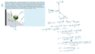# Spring on an inclined plane

• Jpyhsics
In summary, the conversation discusses solving for the maximum distance a well-greased watermelon can be pulled down a ramp without the spring pulling it back up, using the equations F=ma and ΣFx=mgSinθ-kx-μsmgCosθ. The solution is calculated to be 0.253m, taking into account the direction of the friction force opposing the watermelon's potential upward motion on the ramp.f

## Homework Statement

As part of an elaborate prank, a well-greased watermelon (m=4.72 kg) is placed on a ramp inclined with angle θ=27.4° with respect to the horizontal. Because of the grease, the coefficients of static and kinetic friction are µs= 0.560 and µk= 0.392 respectively. The watermelon is attached to the wall, parallel to the ramp, by a spring with constant k=175.2 N/m. What is the farthest distance down the ramp that the watermelon can be pulled away from the spring equilibrium and placed at rest on the surface without the spring pulling the watermelon back up the ramp? Express your answer in metres

F=ma

## The Attempt at a Solution

ΣFx=mgSinθ-kx-μsmgCosθ

Rearranged to make:
x=(mg(μsCosθ-Sinθ))/(-k)
x=-0.00977m

I used the coefficient of static friction, because it was at rest. Does my solution look correct?
I have included an image of my solution as well.

#### Attachments

•IMG_8482D53337F2-1.jpeg
26.6 KB · Views: 318
Hello.

Does a negative answer for x seem right?

Check to see if you have the correct direction for the friction force.

Hello.

Does a negative answer for x seem right?

Check to see if you have the correct direction for the friction force.
Why would force of friction be towards the right?

Why would force of friction be towards the right?
According to the conditions stated in the problem, is the watermelon on the verge of slipping up the slope or slipping down the slope?

According to the conditions stated in the problem, is the watermelon on the verge of slipping up the slope or slipping down the slope?
Slipping up...oh so the friction has to oppose that verge of motion? My revised answer is 0.253m Does that seem right? And thank you so much!

Slipping up...oh so the friction has to oppose that verge of motion? My revised answer is 0.253m Does that seem right? And thank you so much!
Looks right to me.